# Measuring Oral Reading Fluency: A Case for Samejima’s Continuous Response Model

I pitched the idea of using Samejima’s Continuous Response Model (CRM) to measure the Oral Reading Fluency (ORF) a while ago when I published a paper in 2012. In that paper, I used an ORF dataset from the Minneapolis Public Schools District (MPS) as a real data example. Since then, I don’t think anybody has bought the idea of using CRM to measure ORF, so here I am trying one more time with some accessible R code.

Cengiz Zopluoglu (University of Miami)
12-31-2019

I pitched the idea of using Samejima’s Continuous Response Model (CRM) to measure the Oral Reading Fluency (ORF) a while ago when I published a paper as a graduate student. In that paper, I used an ORF dataset from the Minneapolis Public Schools Disctrict (MPS) as a real data example. Since then, I don’t think anybody has bought the idea for using CRM to measure ORF, so here I am trying one more time with some accessible R code.

The ORF dataset from MPS was the only reason I was interested in CRM at all. During my second year in graduate school, I had a chance to complete a semester-long internship in MPS. The task I was assigned was to use IRT for scaling an ORF dataset they had. The dataset had three variables representing the number of words read correctly per minute for three different reading passages. There were 2594 students. Below is a little information about the dataset.

str(wpm)
'data.frame':   2594 obs. of  3 variables:
$spas1wpm: num 66 33 101 14 22 47 67 49 35 41 ...$ spas2wpm: num  74 30 117 12 17 53 58 39 36 42 ...
$spas3wpm: num 74 29 110 11 23 38 71 36 26 45 ... - attr(*, "na.action")= 'omit' Named int [1:276] 13 32 39 50 53 56 105 108 136 152 ... ..- attr(*, "names")= chr [1:276] "13" "32" "39" "50" ... head(wpm)  spas1wpm spas2wpm spas3wpm 1 66 74 74 2 33 30 29 3 101 117 110 4 14 12 11 5 22 17 23 6 47 53 38 require(psych) describe(wpm)[,c("n","mean","sd","min","max","skew","kurtosis")]  n mean sd min max skew kurtosis spas1wpm 2594 63.32 35.44 1 200 0.52 -0.22 spas2wpm 2594 70.09 45.34 3 236 0.84 0.20 spas3wpm 2594 64.93 42.95 2 230 0.86 0.27 cor(wpm)  spas1wpm spas2wpm spas3wpm spas1wpm 1.0000000 0.9396532 0.9375107 spas2wpm 0.9396532 1.0000000 0.9657391 spas3wpm 0.9375107 0.9657391 1.0000000 At the time, I had taken an IRT course and knew a little bit about dichotomous and polytomous IRT models. It was a challenge because the measurement outcomes were kind of continuous. As I didn’t know much about CRM at that time and how to deal with this, I created discrete categories for each variable such that Level 1 (0-50 words), Level 2(51-100 words), Level 3 (101-150 words), Level 4 (151-200 words), and Level 5 (201-250 words). Then, I fit polytomous IRT models and did usual IRT modeling based on the best fitting model. I completed the internship but I was not satisfied as there was so much information thrown away by discretizing. That’s how I ended up exploring and discovering CRM and started working on it. I first briefly introduce the model before demonstrating how to use it for ORF data. ## Continuous Response Model Below is the reparameterized version of the model by Wang and Zeng (1998). In this model, the probability of an individual with a latent parameter $$\theta_i$$ obtaining a score of $$x$$ or higher on an item with three parameters ($$a$$, $$b$$, and $$\alpha$$) is specified as, $P_{ij}(X\geq x|\theta_{i},a_j,b_j,\alpha_j)=\frac{1}{\sqrt{2\pi}}\int_{-\infty}^{v}{e^{-\frac{t^2}{2}}dt}$ $v=a_j\times (\theta_i-b_j-\frac{1}{\alpha_j}ln\frac{x}{k-x})$, where $$k_j$$ is the maximum possible score for the item. The parameter $$a$$ and $$b$$ have a very similar interpretation as in the 2PL IRT model. The $$a$$ parameter controls the steepness, and the $$b$$ parameter controls the location of the operating characteristic curves. There is an extra $$\alpha$$ parameter called “scaling parameter,” and it controls the distance among the curves. Let’s create some plots to see it better. Suppose there is an item with ten categories, and this item has the following parameters: $$a=1$$, $$b=0$$, and $$\alpha=1$$.The figure below displays how the operating characteristic curves look like for this item. Note that there are nine lines in the plot indicating $$P(X\geq 1)$$, $$P(X\geq 2)$$, $$P(X\geq 3)$$, …, $$P(X\geq 9)$$.  theta <- seq(-4,4,.01) a = 1 b = 0 alpha = 1 x <- 1 k <- 10 v <- a*(theta-b-(1/alpha)*log(x/(k-x))) plot(theta,pnorm(v,0,1),type="l",xlim=c(-4,4),ylim=c(0,1), main=expression(paste("a=1, b=0, ",alpha,"=1")), xlab=expression(theta),ylab="Cumulative Probability") for(x in 2:(k-1)){ v <- a*(theta-b-(1/alpha)*log(x/(k-x))) points(theta,pnorm(v,0,1),type="l") }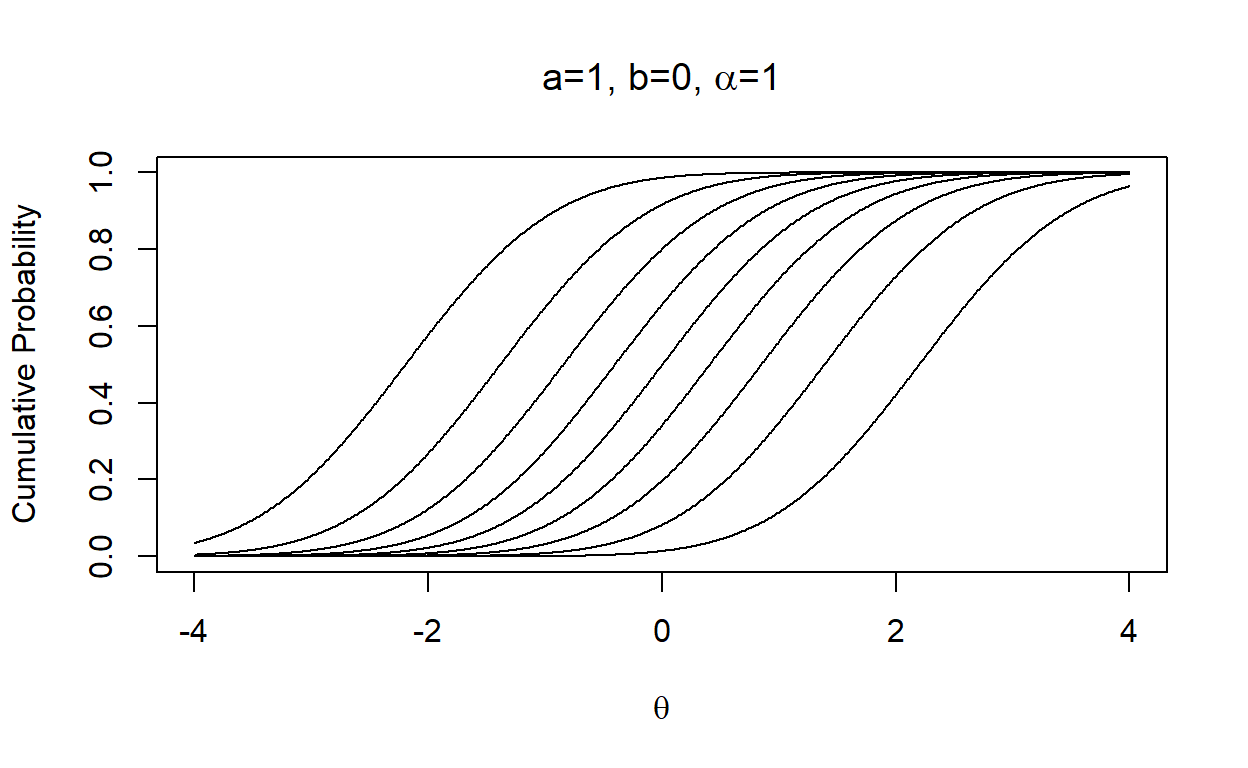Now, let’s keep everything the same but increase the value of the parameter $$a$$ to 3, and we see how steepness changes.  theta <- seq(-4,4,.01) a = 3 b = 0 alpha = 1 x <- 1 k <- 10 v <- a*(theta-b-(1/alpha)*log(x/(k-x))) plot(theta,pnorm(v,0,1),type="l",xlim=c(-4,4),ylim=c(0,1), main=expression(paste("a=3, b=0, ",alpha,"=1")), xlab=expression(theta),ylab="Cumulative Probability") for(x in 2:(k-1)){ v <- a*(theta-b-(1/alpha)*log(x/(k-x))) points(theta,pnorm(v,0,1),type="l") }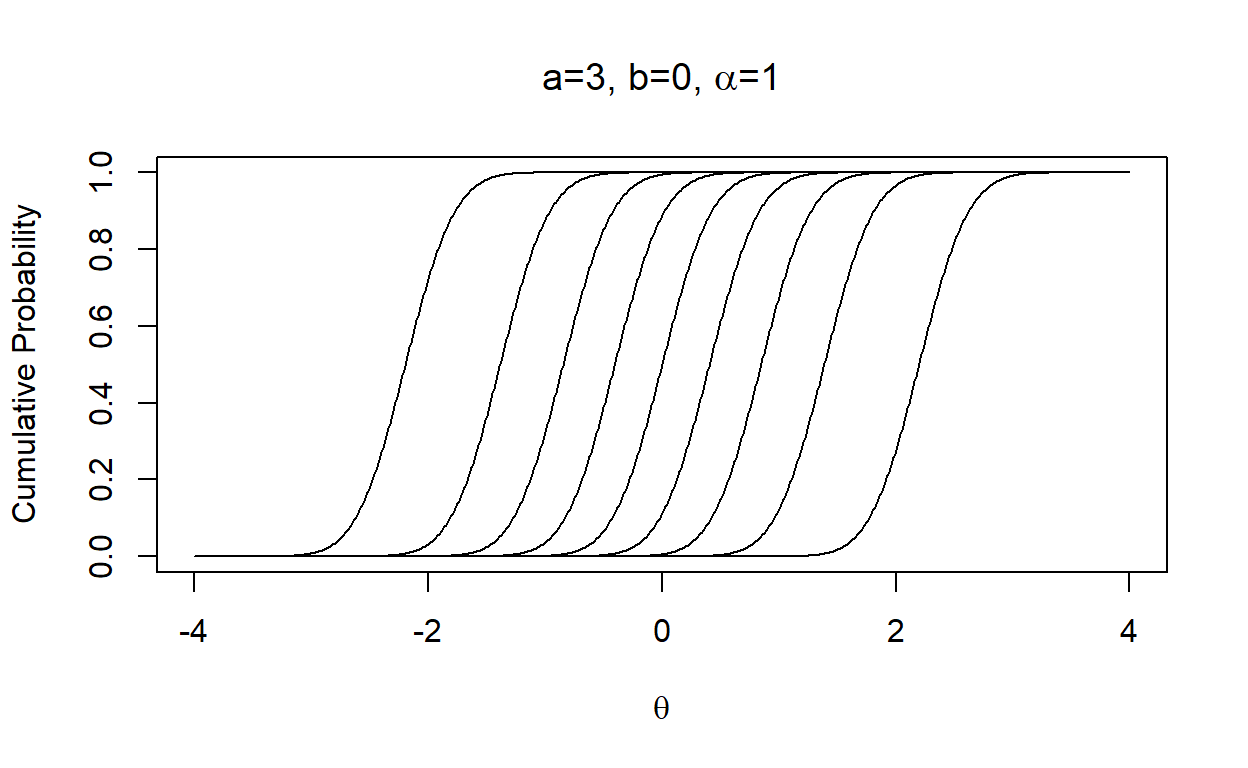Or, we can keep everything the same but change the value of the parameter $$b$$ to -2, and we see the curves shift to left.  theta <- seq(-4,4,.01) a = 1 b = -2 alpha = 1 x <- 1 k <- 10 v <- a*(theta-b-(1/alpha)*log(x/(k-x))) plot(theta,pnorm(v,0,1),type="l",xlim=c(-4,4),ylim=c(0,1), main=expression(paste("a=1, b=-2, ",alpha,"=1")), xlab=expression(theta),ylab="Cumulative Probability") for(x in 2:(k-1)){ v <- a*(theta-b-(1/alpha)*log(x/(k-x))) points(theta,pnorm(v,0,1),type="l") }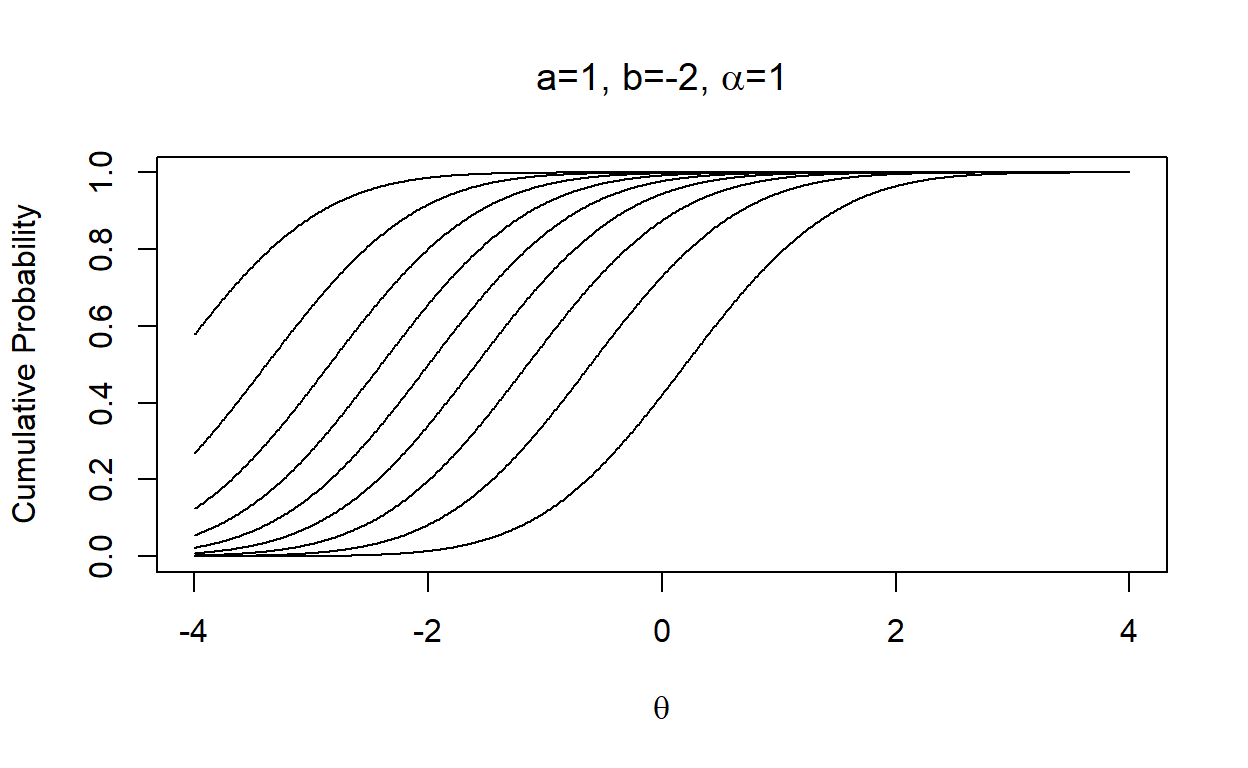Lastly, let’s change the value of the parameter $$\alpha$$ to 4, and we see the distance among curves gets smaller.  theta <- seq(-4,4,.01) a = 1 b = 0 alpha = 4 x <- 1 k <- 10 v <- a*(theta-b-(1/alpha)*log(x/(k-x))) plot(theta,pnorm(v,0,1),type="l",xlim=c(-4,4),ylim=c(0,1), main=expression(paste("a=1, b=0, ",alpha,"=4")), xlab=expression(theta),ylab="Cumulative Probability") for(x in 2:(k-1)){ v <- a*(theta-b-(1/alpha)*log(x/(k-x))) points(theta,pnorm(v,0,1),type="l") }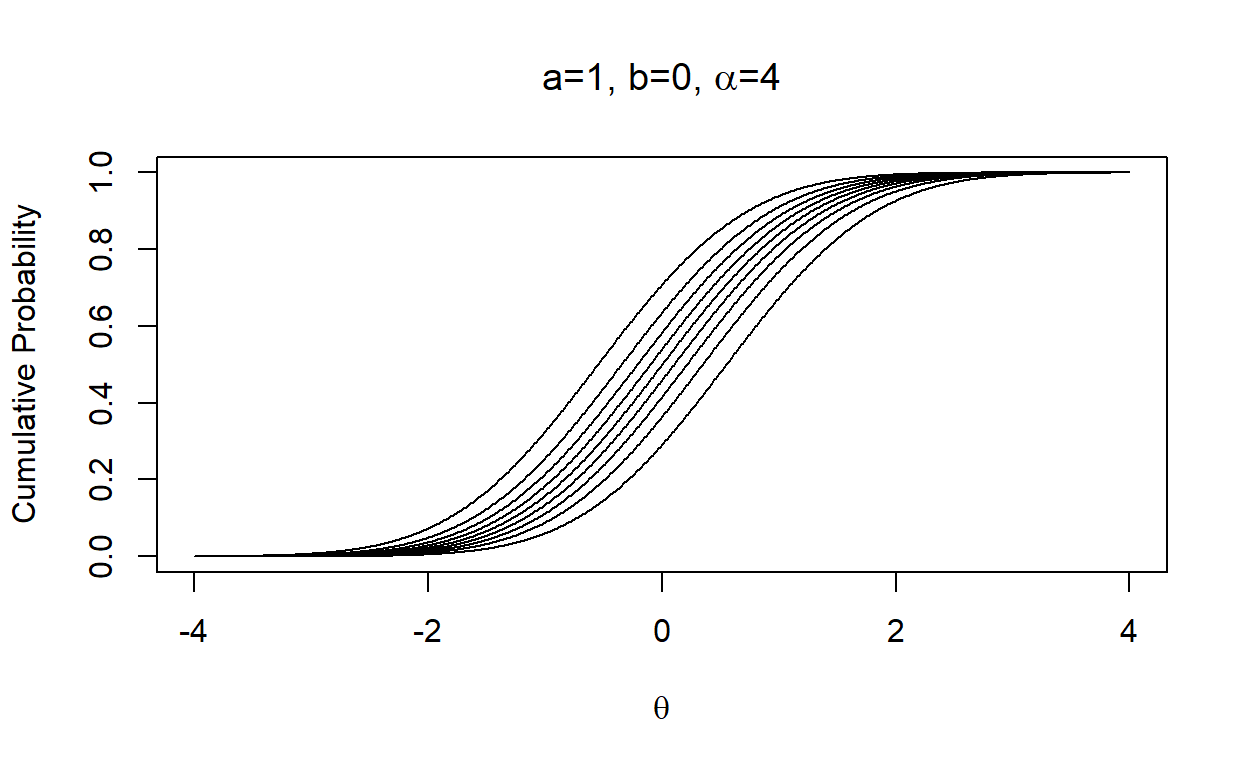For those who are familiar with the Graded Response Model (GRM) may see the difference. In GRM, we estimate a step parameter for each response category, and these step parameters control the location of the operating characteristic curves and hence the distance among the curves at the same time. So, if there is an item with ten categories, GRM estimates nine step parameters. Instead, CRM estimates only two parameters ($$b$$ and $$\alpha$$) per item to control the location and distance among the curves regardless of the number of categories. So, CRM is a restricted version of GRM where we control the distance among the curves with only one parameter. If we think the $$b$$ parameter a kind of common difficulty parameter for an item, the term $\frac{1}{\alpha_j}ln\frac{x}{k-x})$ decides how much each score is away from that common difficulty after accounting for the distance between the score and the maximum possible score. Wang & Zeng (1998) also simplifies the equation by transforming the observed response (this is like a logit transformation for the observed responses), $Z=ln\frac{X}{k-X}$ and provides the conditional PDF of Z as $f(z|\theta_i,a_j,b_j,\alpha_j)=\frac{a}{\alpha\sqrt{2\pi}}e^\frac{-(a_j(\theta_i-b_j-\frac{z_i}{\alpha_j}))^2}{2}$, which is a normal density function with a mean of $$\alpha_j(\theta_i - b_j)$$ and standard deviation of $$\alpha_j/a_j$$. ## Formatting Data for Stan There are other tools we can use to fit CRM. I will focus here fitting the model using Stan, but I also provide two other tools at the very end as supplemental material. Before introducing the Stan model syntax, I should first reformat data as this is the only way to run Stan with missing data. Although I don’t have missing data in the ORF dataset used for this blog post, I force myself to use this procedure to get used to it. # Wide format data (2594 x 3) with rows representing a different individual and # columns represent a different outcome head(wpm)  spas1wpm spas2wpm spas3wpm 1 66 74 74 2 33 30 29 3 101 117 110 4 14 12 11 5 22 17 23 6 47 53 38 dim(wpm)  2594 3 # add an arbitrary individual id variable wpm$id <- 1:nrow(wpm)

# Convert the wide format data to long format (7782 x 3)
# This is like repeated measures data

wpm.long <- reshape(data      = wpm,
idvar       = "id",
varying     = list(colnames(wpm)[1:3]),
timevar     = "Item",
times       = 1:3,
v.names      = "score",
direction   = "long")

wpm.long <- wpm.long[order(wpm.long$id),] head(wpm.long)  id Item score 1.1 1 1 66 1.2 1 2 74 1.3 1 3 74 2.1 2 1 33 2.2 2 2 30 2.3 2 3 29 dim(wpm.long)  7782 3  # Each individual is represented in three rows, one for each item # There are extra two columns for item and person id # The next line doesn't change anything for this case as there is no # missing data but this is critical step if you have missing data wpm.long <- na.omit(wpm.long) # Transform the observed scores to logits(from X to Z in the above description) # This assumes each reading passage has 250 words so the # maximum possible score is 250 wpm.long$score <- log(wpm.long$score/(250-wpm.long$score))

head(wpm.long)
    id Item      score
1.1  1    1 -1.0252810
1.2  1    2 -0.8664189
1.3  1    3 -0.8664189
2.1  2    1 -1.8833898
2.2  2    2 -1.9924302
2.3  2    3 -2.0308669
# Create each column in the long format data as separate vectors
# These are what we will feed to Stan as our data

id            <- wpm.long$id item <- wpm.long$Item
score         <- wpm.long$score n_obs <- length(wpm.long) ## Stan Model Syntax Below is the Stan model syntax for fitting CRM. I follow the guidelines in the Stan manual and imposes weakly-informative prior on model parameters. crm <- ' data{ int J; // number of items int I; // number of individuals int n_obs; // number of observed responses int item[n_obs]; // item id int id[n_obs]; // person id real Y[n_obs]; // vector of transformed outcome } parameters { vector[J] b; // vector of b parameters forJ items real mu_b; // mean of the b parameters real<lower=0> sigma_b; // standard dev. of the b parameters vector<lower=0>[J] a; // vector of a parameters for J items real mu_a; // mean of the a parameters real<lower=0> sigma_a; // standard deviation of the a parameters vector<lower=0>[J] alpha; // vector of alpha parameters for J items real mu_alpha; // mean of alpha parameters real<lower=0> sigma_alpha; // standard deviation of alpha parameters vector[I] theta; // vector of theta parameters for I individuals } model{ mu_b ~ normal(0,5); sigma_b ~ cauchy(0,2.5); b ~ normal(mu_b,sigma_b); mu_a ~ normal(0,5); sigma_a ~ cauchy(0,2.5); a ~ normal(mu_a,sigma_a); mu_alpha ~ normal(0,5); sigma_alpha ~ cauchy(0,2.5); alpha ~ normal(mu_alpha,sigma_alpha); theta ~ normal(0,1); // The mean and variance of theta is fixed to 0 and 1 // for model identification for(i in 1:n_obs) { Y[i] ~ normal(alpha[item[i]]*(theta[id[i]]-b[item[i]]),alpha[item[i]]/a[item[i]]); } } ' ## Fitting the Model The code below fits the model with four chains and 50,000 iterations for each chain. By default, the first 50% of the iterations are used as warm-up and the remaining 50% of the iterations used for inference. require(rstan) data <- list(I = 2594, J = 3, n_obs = 7782, id = id, item = item, Y = score) fit_crm <- stan(model_code = crm, data = data, iter = 50000, chains = 4, control = list(max_treedepth = 15,adapt_delta=0.9)) print(get_elapsed_time(fit_crm))  warmup sample chain:1 1227.35 1882.09 chain:2 1166.93 1942.92 chain:3 1273.22 1944.18 chain:4 1280.11 2007.22 sum(get_elapsed_time(fit_crm))/3600 # how many hours it took to run the model with the   3.53445  # above settings ## Model Convergence print(fit_crm, pars = c("b","mu_b","sigma_b", "a","mu_a","sigma_a", "alpha","mu_alpha","sigma_alpha"), probs = c(0.025,0.975), digits = 3) Inference for Stan model: ebc8a1e0d17f738279125cd2fac99954. 4 chains, each with iter=50000; warmup=25000; thin=1; post-warmup draws per chain=25000, total post-warmup draws=1e+05. mean se_mean sd 2.5% 97.5% n_eff Rhat b 1.519 0.000 0.031 1.457 1.579 4892 1.001 b 1.130 0.000 0.026 1.078 1.180 4295 1.001 b 1.269 0.000 0.027 1.215 1.323 4421 1.001 mu_b 1.299 0.003 0.467 0.377 2.181 31652 1.000 sigma_b 0.571 0.004 0.671 0.121 2.311 28645 1.000 a 3.201 0.001 0.075 3.057 3.350 15234 1.000 a 5.164 0.001 0.175 4.835 5.521 17507 1.000 a 4.604 0.001 0.136 4.347 4.880 18256 1.000 mu_a 4.107 0.005 1.164 1.424 6.176 57866 1.000 sigma_a 1.762 0.006 1.356 0.573 5.187 44796 1.000 alpha 0.825 0.000 0.013 0.801 0.850 7178 1.000 alpha 1.008 0.000 0.015 0.980 1.038 6663 1.000 alpha 0.997 0.000 0.015 0.969 1.026 6645 1.000 mu_alpha 0.936 0.003 0.338 0.329 1.505 16201 1.000 sigma_alpha 0.359 0.004 0.521 0.063 1.672 19521 1.000 Samples were drawn using NUTS(diag_e) at Wed Feb 23 16:29:05 2022. For each parameter, n_eff is a crude measure of effective sample size, and Rhat is the potential scale reduction factor on split chains (at convergence, Rhat=1). It seems that we have convergence, and all chains yield consistent results as all $$\hat{R}$$ values are less than 1.01, The figures below are the posterior distributions for all these parameters. They all look fine. plot(fit_crm, plotfun = "hist", pars = c("b","b","b", "a","a","a", "alpha","alpha","alpha"), inc_warmup = FALSE)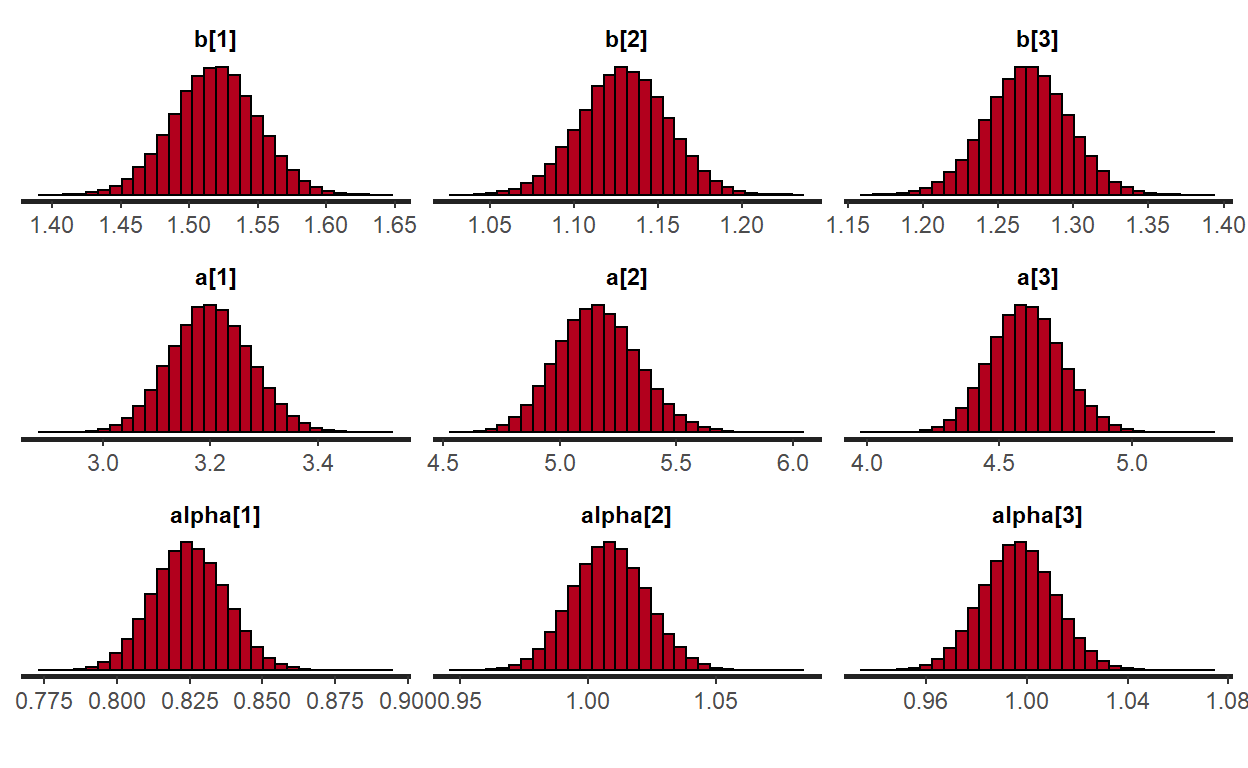I also used the recommendations provided at this link to check some other things (seemed important). None of them indicated any red flag about the model convergence. source("https://raw.githubusercontent.com/betanalpha/knitr_case_studies/master/qr_regression/stan_utility.R") check_treedepth(fit_crm,15)  "0 of 100000 iterations saturated the maximum tree depth of 15 (0%)" check_energy(fit_crm)  "E-BFMI indicated no pathological behavior" check_div(fit_crm)  "0 of 100000 iterations ended with a divergence (0%)" # Parameter Interpretation b <- summary(fit_crm)$summary[1:3,1]

b
    b     b     b
1.518776 1.129768 1.269264 
a     <- summary(fit_crm)$summary[6:8,1] a  a a a 3.201176 5.164072 4.604099  alpha <- summary(fit_crm)$summary[11:13,1]

alpha
 alpha  alpha  alpha
0.8249487 1.0083558 0.9966818 
thetas = summary(fit_crm)$summary[16:2609,1] The $$b$$ parameters for three reading passages in this dataset were 1.52, 1.13, 1.27. I think it is fair to call them “reading passage difficulty” in this context. Given that we fix the mean and variance of the latent person parameter ($$\theta$$) to 0 and 1, we can compare the passage difficulty relative to the levels of individuals. Note that $$\theta$$ represents here a latent variable indicating oral reading fluency. So, these three reading passages seem very difficult compared to the ORF levels of these individuals. The $$a$$ parameters were 3.2, 5.16, 4.6. We can call them “reading passage discrimination” parameters. So, these reading passages are highly informative about the latent construct being measured. These very high discrimination parameters are not very surprising, given that the correlations among the three variables were 0.94, 0.94, and 0.96. The $$\alpha$$ parameters were 0.82, 1.01, 1. I think it would be wise to go back and fix all the $$\alpha$$ parameters to 1 in the model and then re-fit, but I will not do it. Note that the $$\alpha$$ scales the distance between the response categories. We can plot the operating characteristic curves, but it would be too messy for all 250 score categories, However, I can draw it for $$P(X\geq 50)$$, $$P(X\geq 100)$$, $$P(X\geq 150)$$, …, $$P(X\geq 200)$$. Below is the plot for Item 1.  theta <- seq(-4,4,.01) a1 = 3.20 b1 = 1.52 alpha1 = 0.83 x <- 50 k <- 250 v <- a1*(theta-b1-(1/alpha1)*log(x/(k-x))) plot(theta,pnorm(v,0,1),type="l",xlim=c(-2,4),ylim=c(0,1), main=expression(paste("a=3.2, b=1.5, ",alpha,"= 0.8")), xlab=expression(theta),ylab="Cumulative Probability") for(x in c(100,150,200)){ v <- a1*(theta-b1-(1/alpha1)*log(x/(k-x))) points(theta,pnorm(v,0,1),type="l") } abline(h=.5,lty=2) text(-0.2,.8,"P(X>50)",cex=0.8) text(0.95,.8,"P(X>100)",cex=0.8) text(1.95,.8,"P(X>150)",cex=0.8) text(3.1,.8,"P(X>200)",cex=0.8)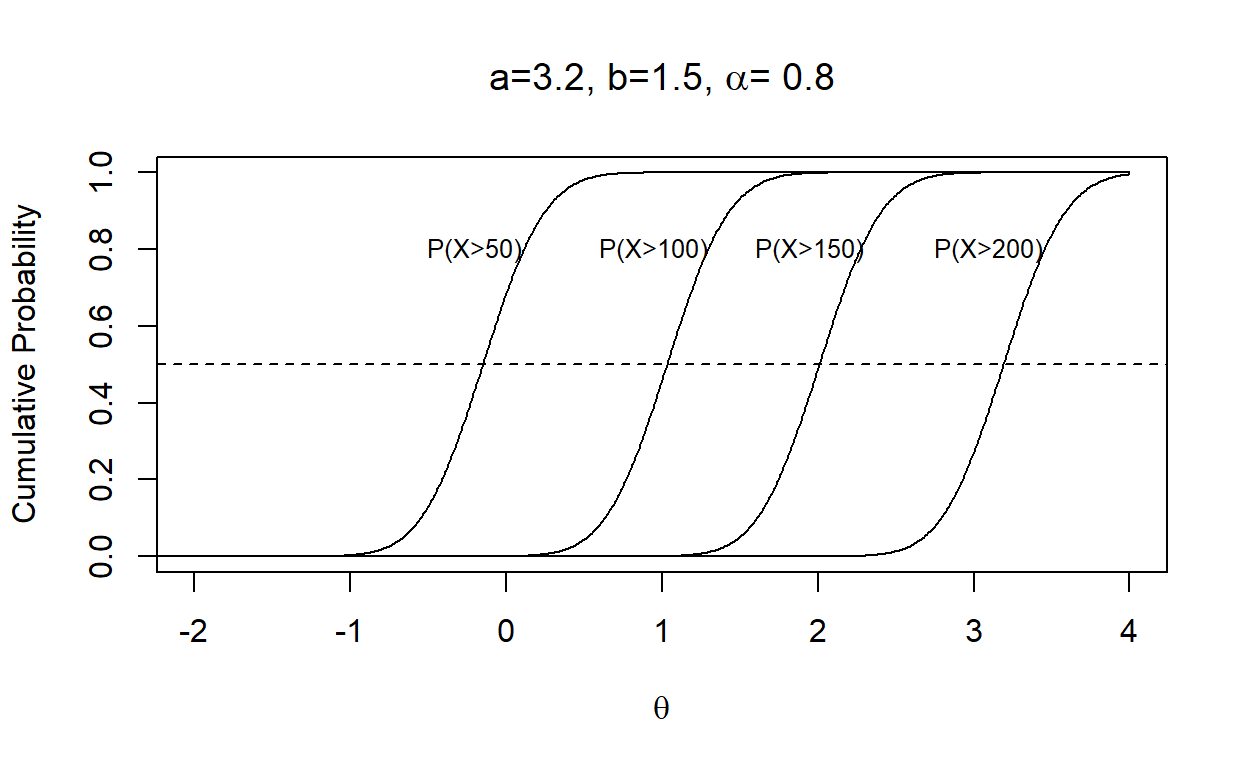Let’s check the latent person parameter estimates. thetas = summary(fit_crm)$summary[16:2609,1]

psych::describe(thetas)
   vars    n mean   sd median trimmed  mad   min  max range skew
X1    1 2594    0 0.99   0.05       0 1.07 -2.55 3.45  6.01 0.05
kurtosis   se
X1     -0.4 0.02
hist(thetas)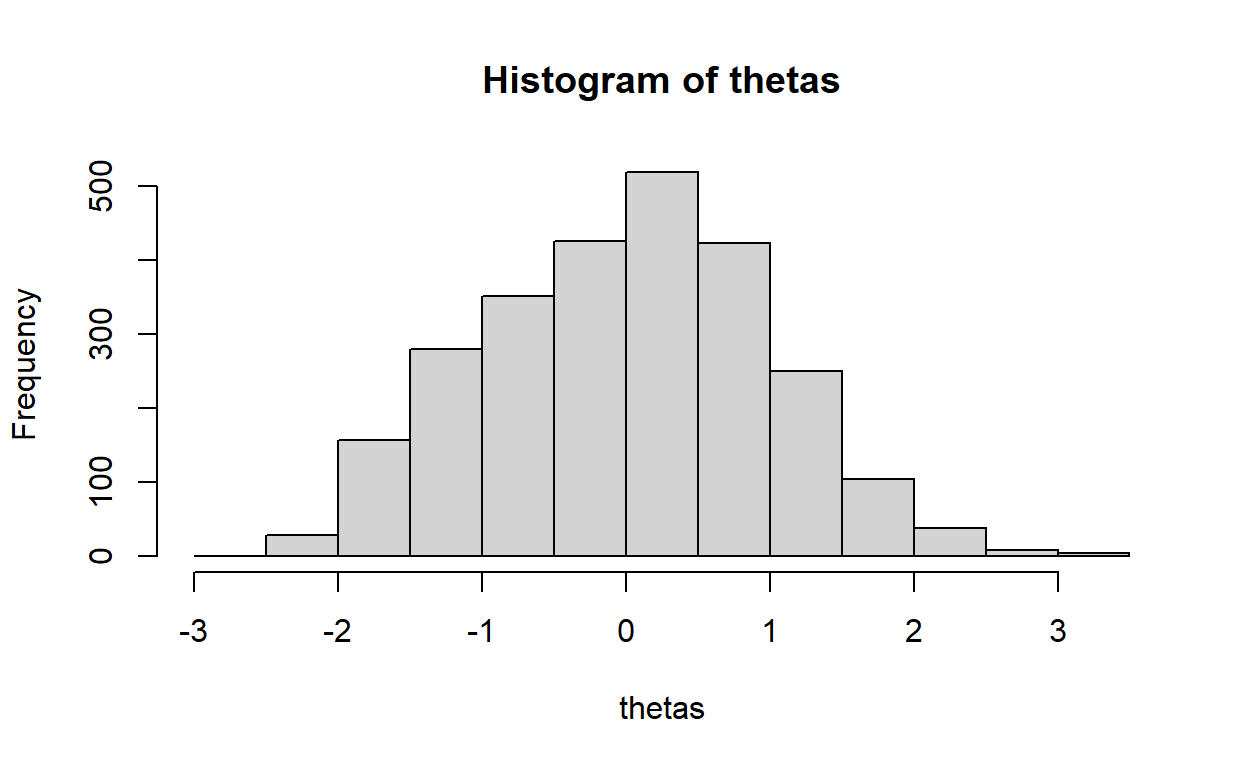As expected, the estimates have a mean of 0 and a standard deviation of .99.

Lower estimates indicate lower levels of oral reading fluency, and higher estimates indicate higher levels of oral reading fluency.

We can have a better understanding if we check a few individuals.

low = order(thetas,decreasing=F)[1:5]
high = order(thetas,decreasing=T)[1:5]

# The individuals with the highest oral reading fluency and their reading scores

cbind(wpm[high,],thetas[high])
     spas1wpm spas2wpm spas3wpm   id thetas[high]
1472      200      234      221 1298     3.451058
800       183      236      212  708     3.321318
2395      176      234      210 2165     3.207132
1266      159      227      227 1108     3.193841
2308      181      224      220 2084     3.111148
# The individuals with the lowest oral reading fluency and their reading scores

cbind(wpm[low,],thetas[low])
     spas1wpm spas2wpm spas3wpm   id thetas[low]
2349       18        6        3 2121   -2.554590
1254       11        7        4 1097   -2.488763
666         2        3       34  584   -2.423383
1101       12        6        8  957   -2.284357
502        11        7        7  437   -2.282513

For instance, the model provides an estimate of 3.11 for a student who had read 181 words, 224 words, and 220 words and an estimate of -2.28 for a student who had read 12 words, six words, and eight words in the three reading passages, respectively. We can check how the estimated theta is related to the scores from each reading passage for all examinees.

plot(thetas,wpm[,1],xlab=expression(theta),ylab="Word per minute",main="Reading Passage 1")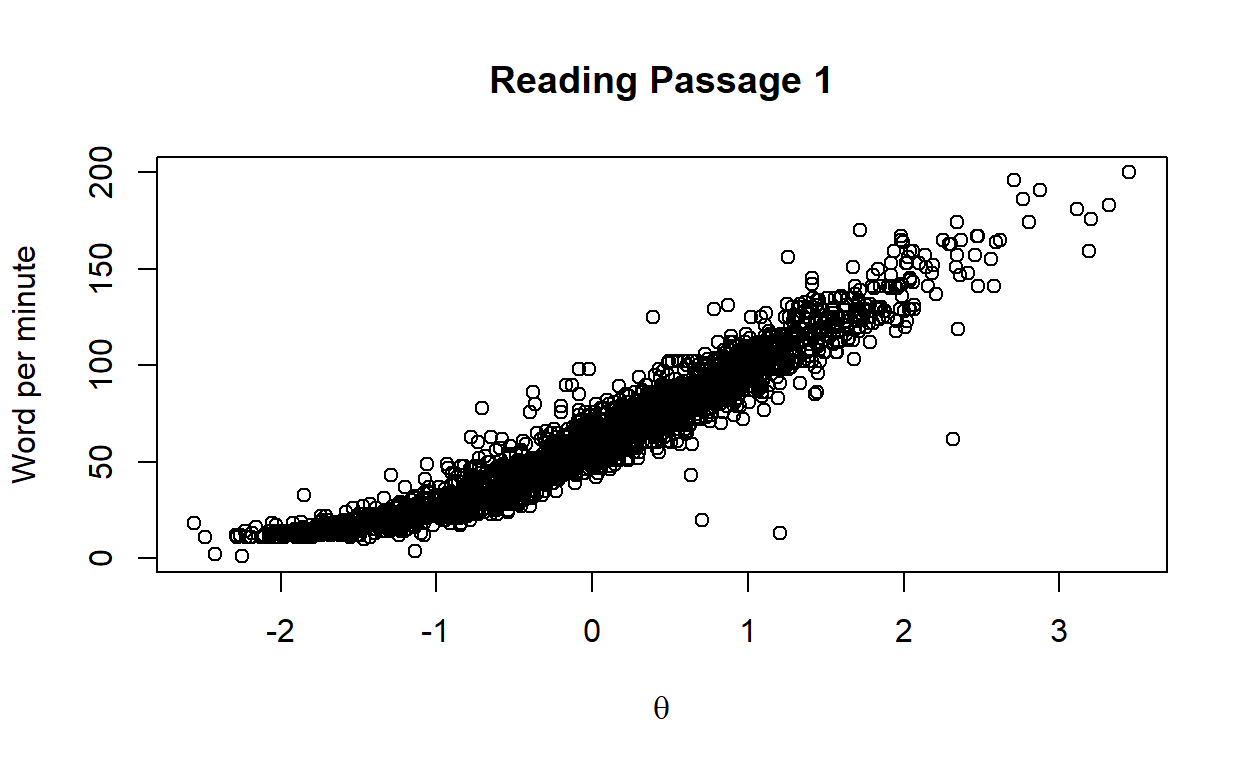plot(thetas,wpm[,2],xlab=expression(theta),ylab="Word per minute",main="Reading Passage 2")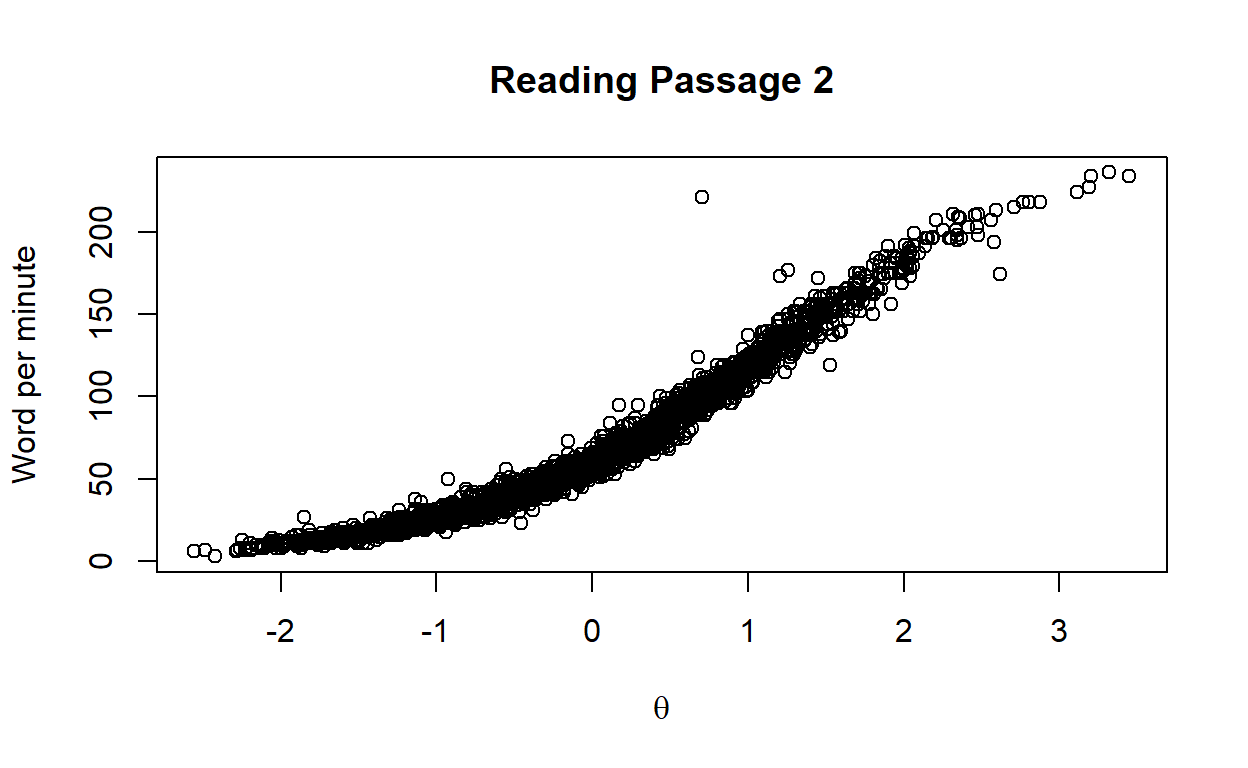plot(thetas,wpm[,3],xlab=expression(theta),ylab="Word per minute",main="Reading Passage 3")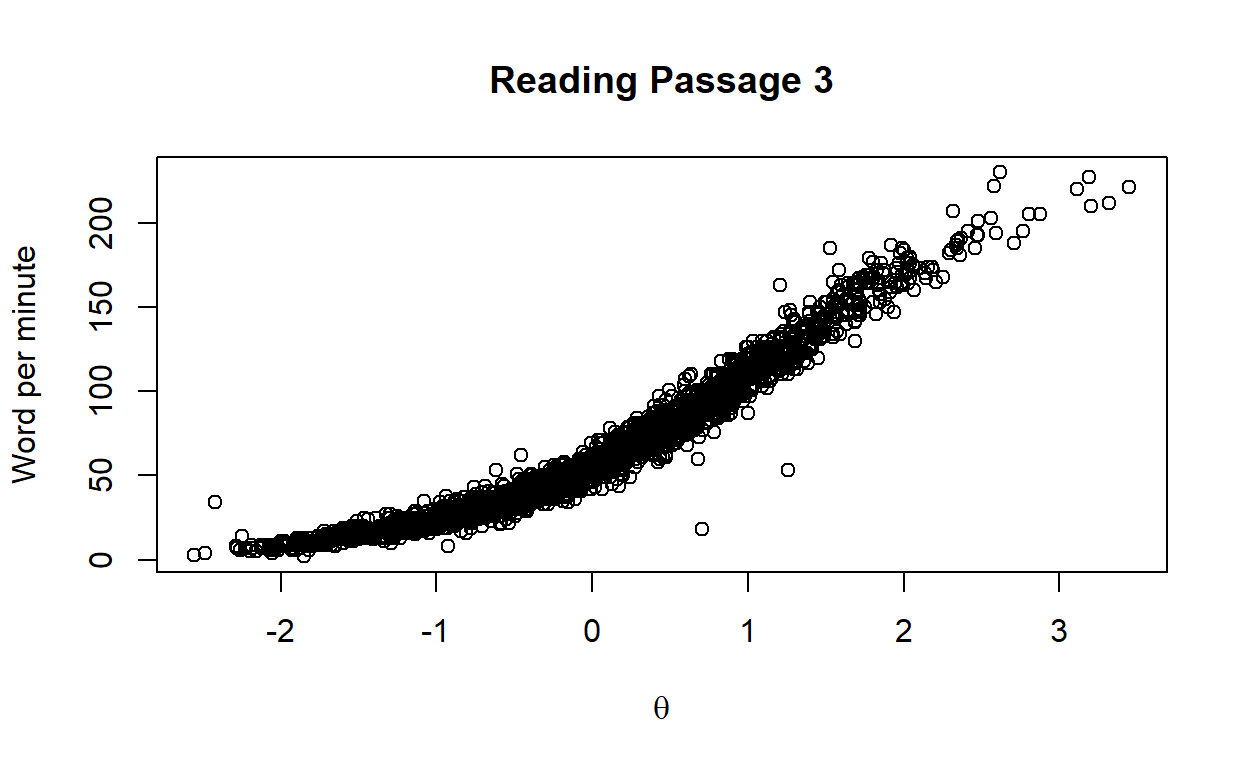It is not surprising to see that there is a strong relationship because of the high discrimination parameters. Note that the relationship is not linear as we model the relationship between $$\theta$$ and $$ln\frac{x}{k-x}$$

# Model Fit Assessment

The first thing I would like to look at is how well the model predicts the observed ORF scores. Treating the item and person parameter estimates as known, we can calculate a predicted score on each item for each individual as well as a standardized residual.

Note that I mentioned before that the conditional PDF of Z is a normal density function with a mean of $$\alpha_j(\theta_i - b_j)$$ and standard deviation of $$\alpha_j/a_j$$, where Z is defined as $ln\frac{X}{k-X}$.

# Remember the data with observed outcomes

head(wpm.long)
    id Item      score
1.1  1    1 -1.0252810
1.2  1    2 -0.8664189
1.3  1    3 -0.8664189
2.1  2    1 -1.8833898
2.2  2    2 -1.9924302
2.3  2    3 -2.0308669
# For each observation, compute the model prediction

wpm.long$pred <- NA wpm.long$sres <- NA

for(i in 1:nrow(wpm.long)){

wpm.long[i,]$pred = alpha[wpm.long[i,2]]*(thetas[wpm.long[i,1]]-b[wpm.long[i,2]]) wpm.long[i,]$sres = (wpm.long[i,]$score-wpm.long[i,]$pred)/(alpha[wpm.long[i,2]]/a[wpm.long[i,2]])

}

head(wpm.long)
    id Item      score       pred        sres
1.1  1    1 -1.0252810 -0.9947824 -0.11834838
1.2  1    2 -0.8664189 -0.8236896 -0.21882874
1.3  1    3 -0.8664189 -0.9531861  0.40081459
2.1  2    1 -1.8833898 -1.9047571  0.08291486
2.2  2    2 -1.9924302 -1.9359749 -0.28912342
2.3  2    3 -2.0308669 -2.0525941  0.10036738

Note that the observed values and predicted values are on a logit metric because of the transformation. Let’s check the correlation between observed outcomes and model predicted outcomes for each item.

cor(wpm.long[wpm.long$Item==1,c("score","pred")])  score pred score 1.0000000 0.9625937 pred 0.9625937 1.0000000 plot(wpm.long[wpm.long$Item==1,c("score","pred")])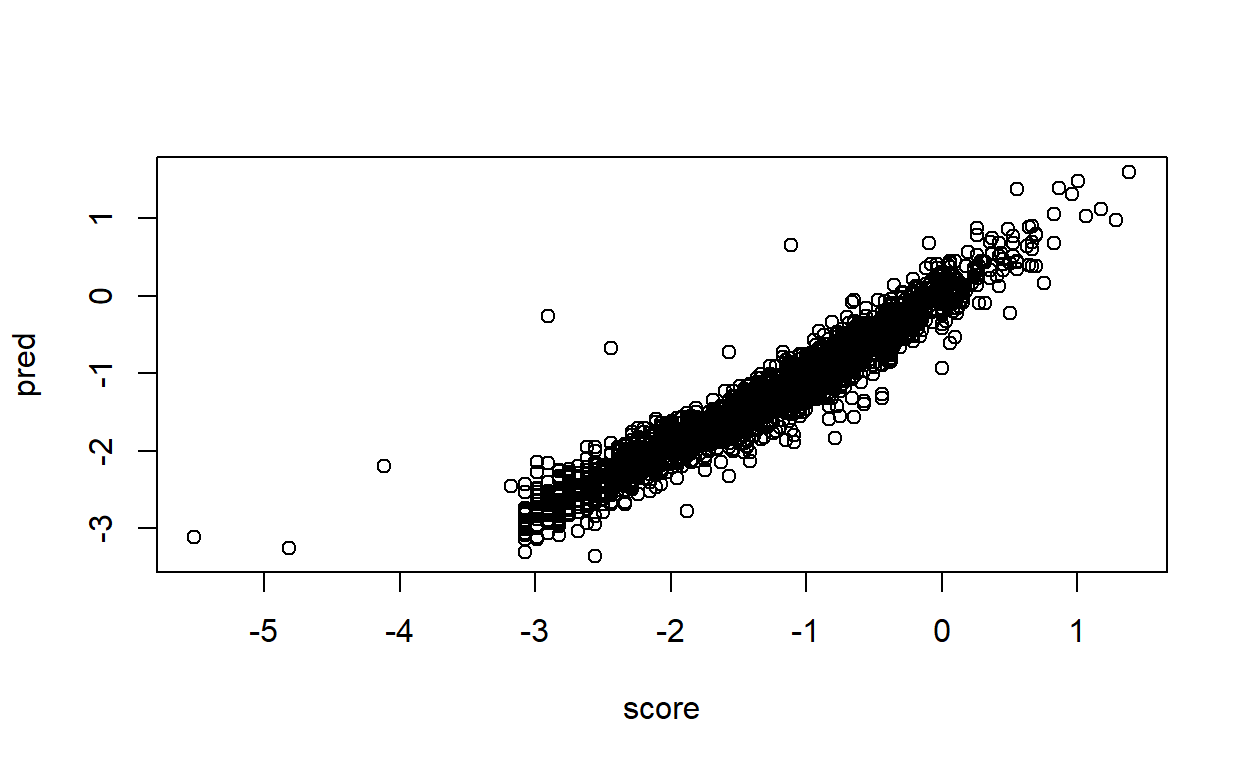cor(wpm.long[wpm.long$Item==2,c("score","pred")])  score pred score 1.0000000 0.9901743 pred 0.9901743 1.0000000 plot(wpm.long[wpm.long$Item==2,c("score","pred")])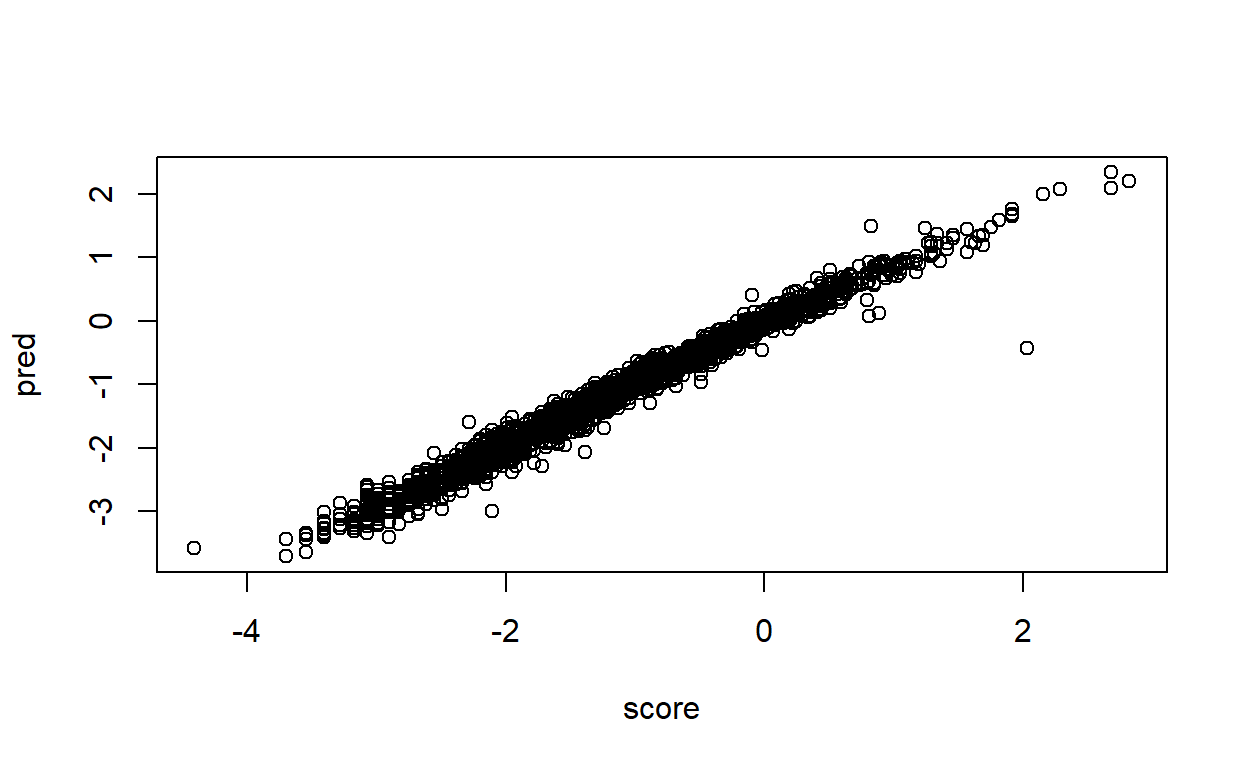cor(wpm.long[wpm.long$Item==3,c("score","pred")])  score pred score 1.0000000 0.9855805 pred 0.9855805 1.0000000 plot(wpm.long[wpm.long$Item==3,c("score","pred")])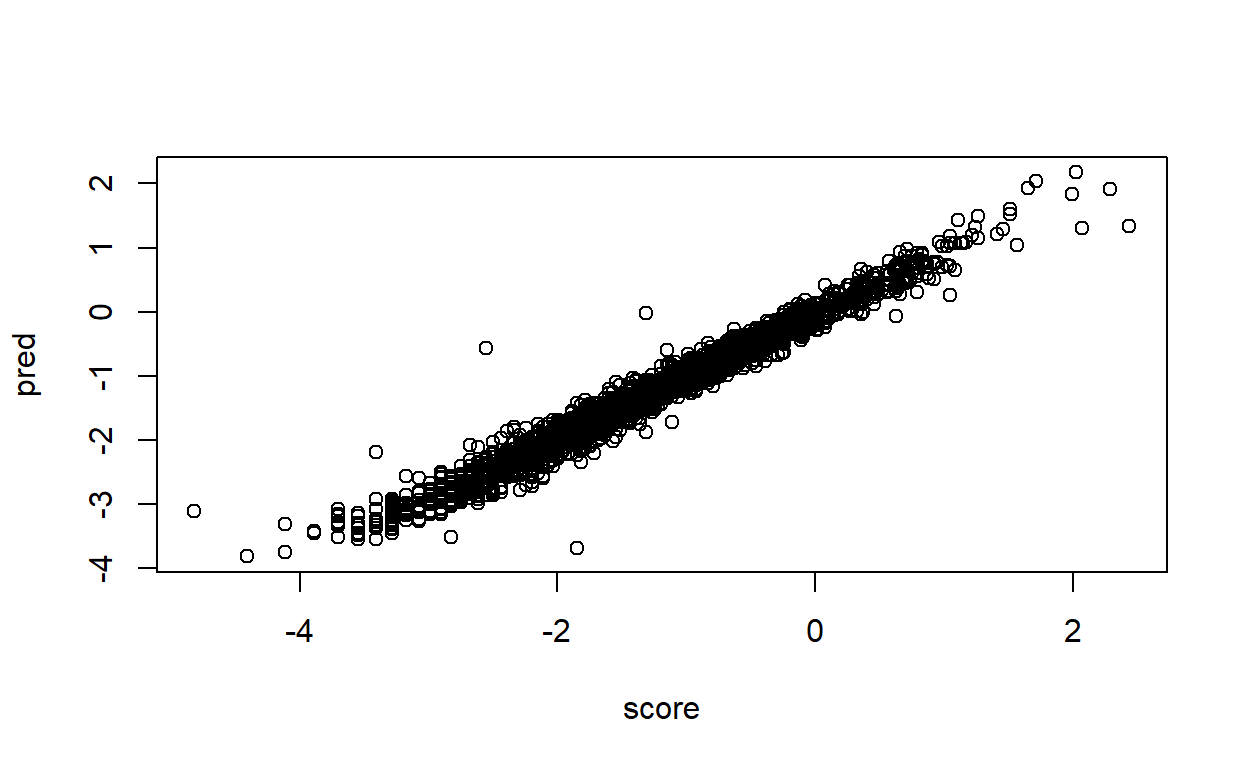It looks like the model predicts the observed outcomes pretty well.

We can also check if there is any systematic relationship between residuals and the latent variable.

plot(thetas,wpm.long[wpm.long$Item==1,c("sres")])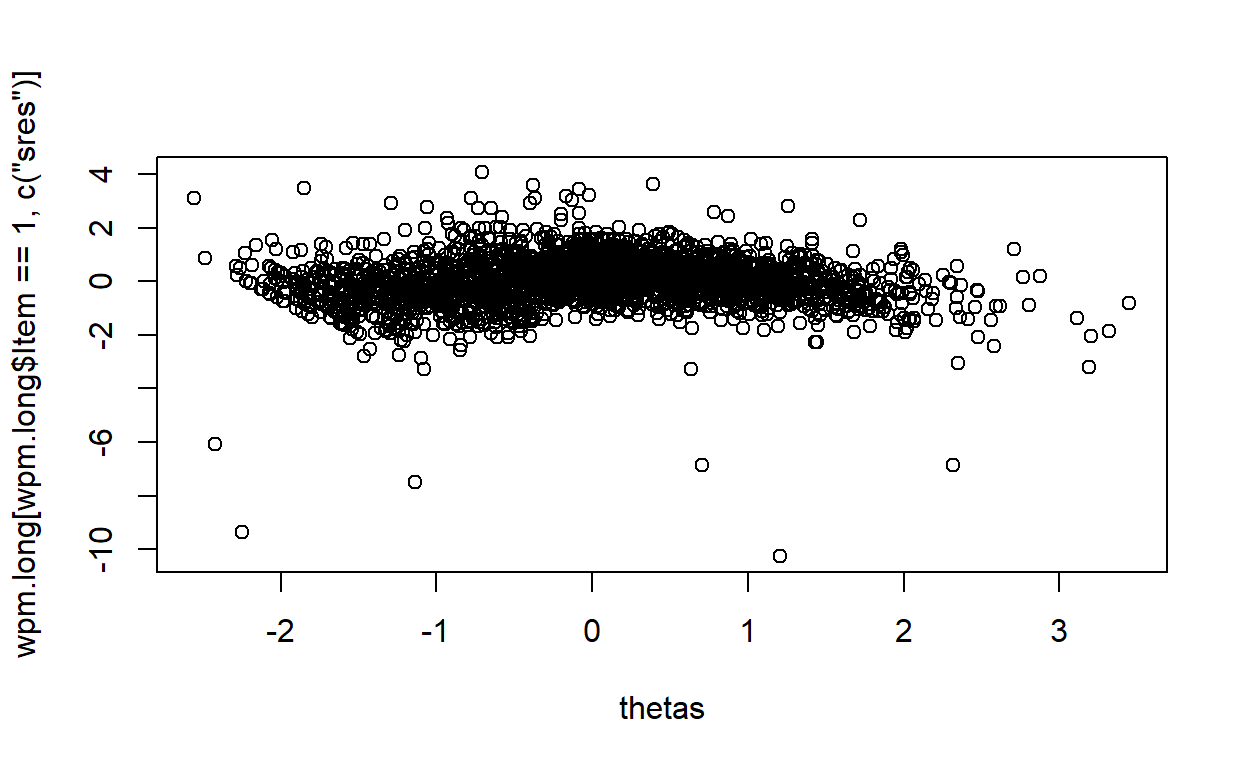plot(thetas,wpm.long[wpm.long$Item==2,c("sres")])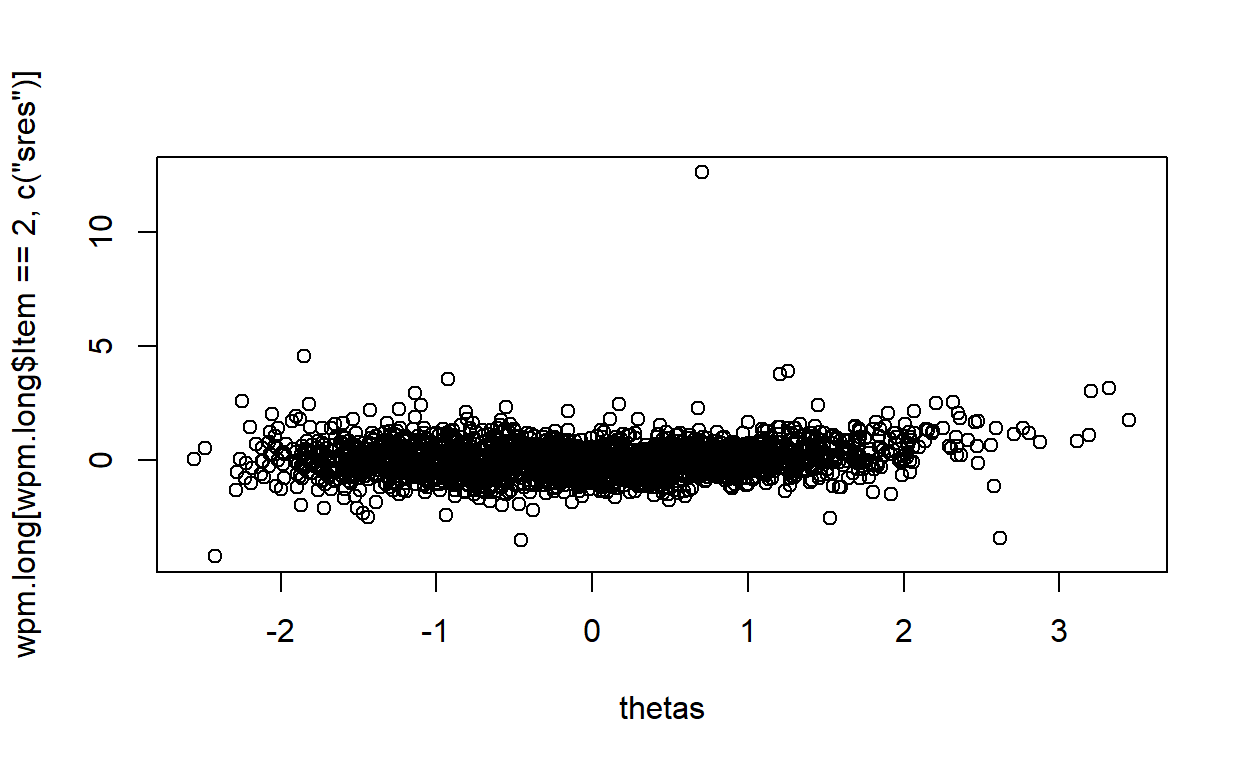plot(thetas,wpm.long[wpm.long$Item==3,c("sres")])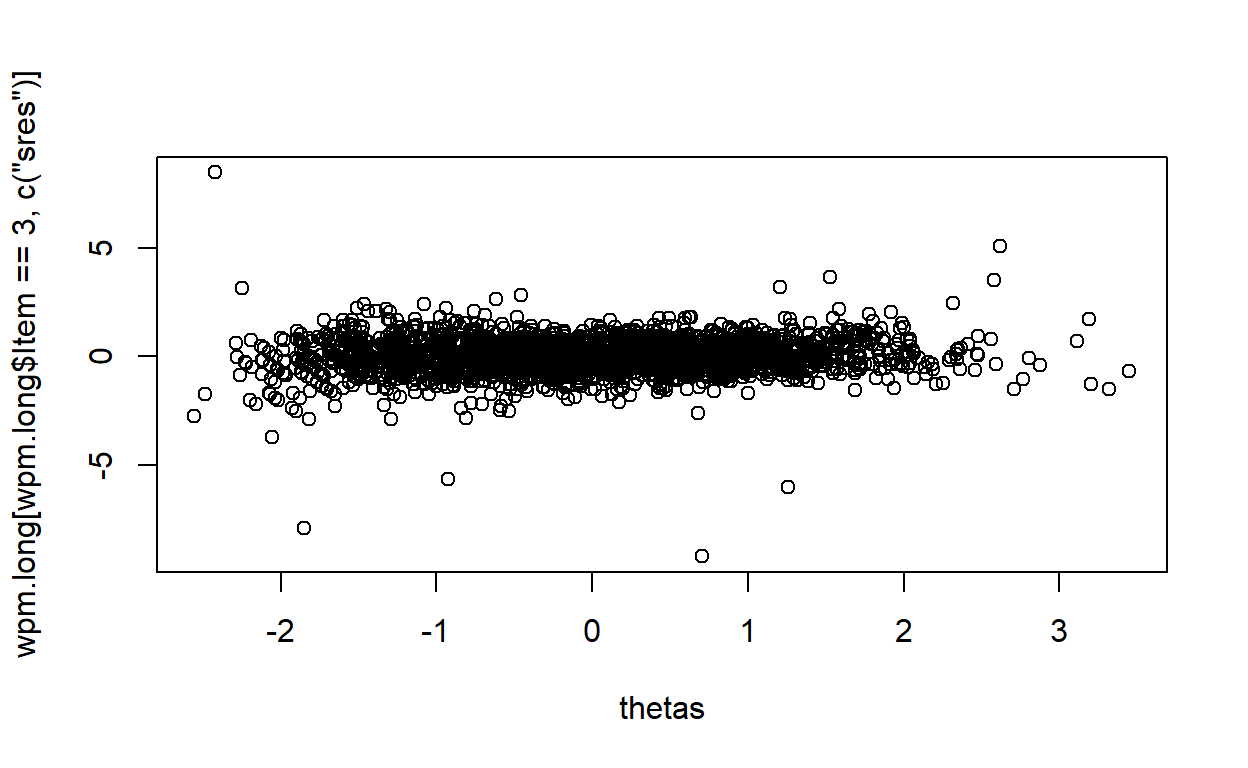There are some individuals with extreme residuals for each item; however, there doesn’t seem to be any systematic pattern between residuals and the latent variable. We can also check the distribution of standardized residuals within each item. psych::describeBy(wpm.long$sres,wpm.long$Item)  Descriptive statistics by group group: 1 vars n mean sd median trimmed mad min max range skew X1 1 2594 0 0.91 0.04 0.01 0.76 -10.26 4.06 14.32 -1.49 kurtosis se X1 15.59 0.02 ---------------------------------------------------- group: 2 vars n mean sd median trimmed mad min max range skew X1 1 2594 0 0.74 -0.01 -0.01 0.63 -4.24 12.61 16.85 2.14 kurtosis se X1 34.15 0.01 ---------------------------------------------------- group: 3 vars n mean sd median trimmed mad min max range skew X1 1 2594 0 0.8 0.02 0.01 0.66 -9.2 8.46 17.66 -0.71 kurtosis se X1 18.05 0.02 hist(wpm.long[wpm.long$Item==1,]$sres)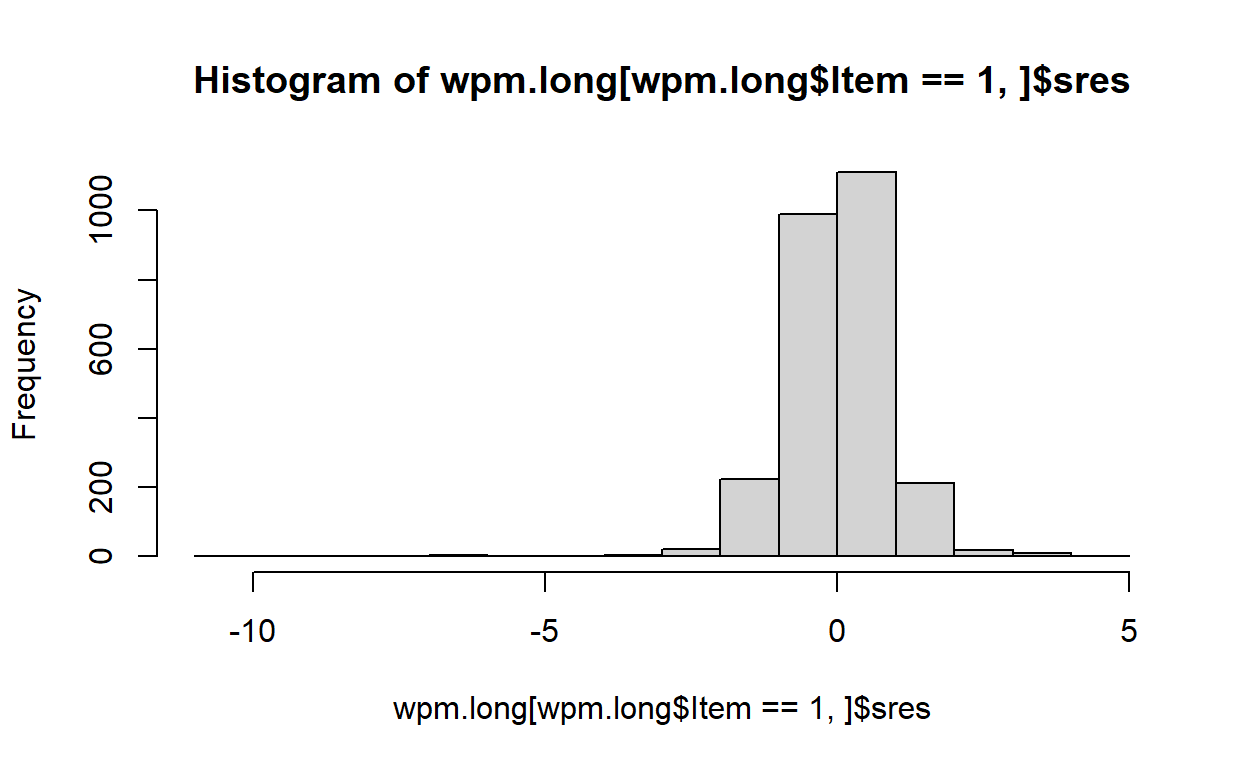hist(wpm.long[wpm.long$Item==2,]$sres)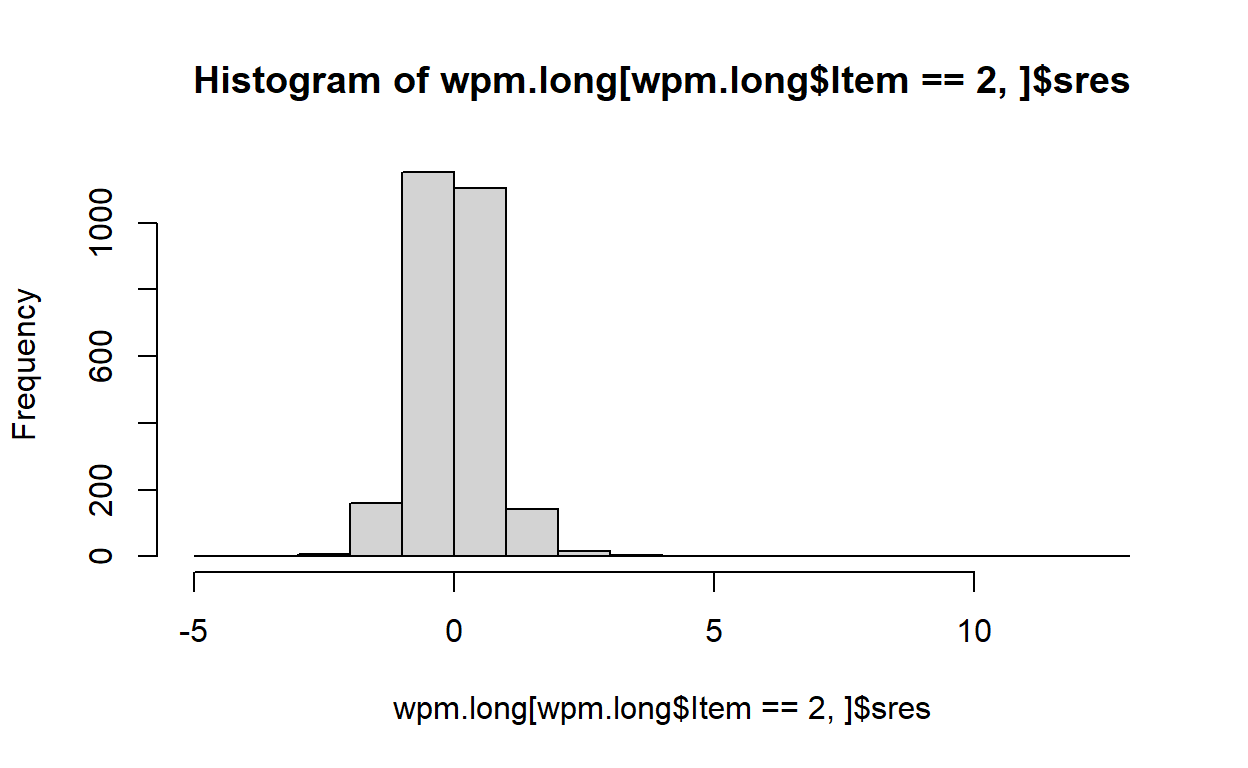hist(wpm.long[wpm.long$Item==3,]$sres)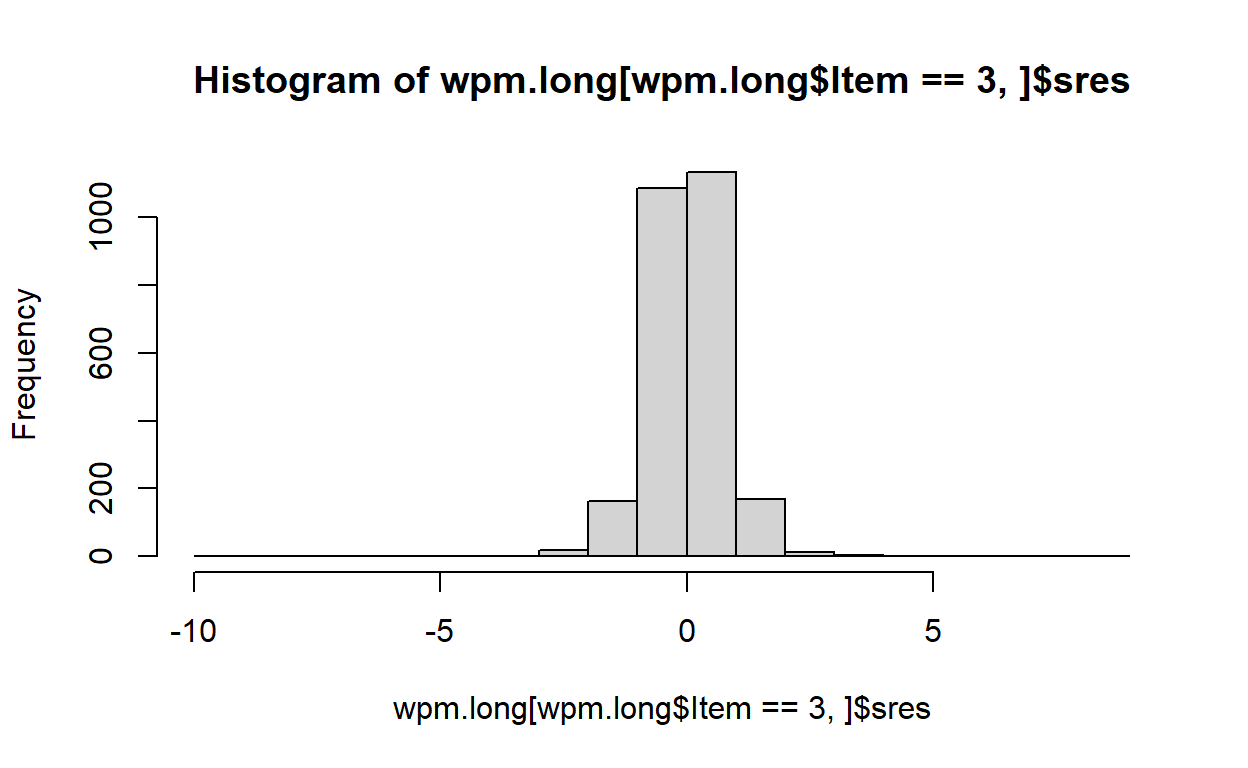There are some extreme residuals we may want to look deeper. So, let’s filter the observations with a standardized residual outside of the range [-4,4] and look at their response pattern. outliers = which(abs(wpm.long$sres)>4)

length(outliers)
 16
wpm.long[outliers,]
         id Item      score        pred       sres
386.2   386    2 -2.1113349 -3.00178168   4.560227
386.3   386    3 -4.8202816 -3.10606181  -7.918713
584.1   584    1 -4.8202816 -3.25207870  -6.085341
584.2   584    2 -4.4107760 -3.58284045  -4.240089
584.3   584    3 -1.8489179 -3.68039351   8.460367
591.1   591    1 -5.5174529 -3.10658824  -9.355252
604.1   604    1 -4.1190372 -2.19143516  -7.479973
919.1   919    1 -2.4423470 -0.67317488  -6.865193
919.2   919    2  2.0308669 -0.43058046  12.605759
919.3   919    3 -2.5563656 -0.56462807  -9.200686
934.3   934    3 -3.4094962 -2.18899591  -5.638012
1154.3 1154    3  2.4423470  1.34338758   5.076563
1911.1 1911    1 -1.1093076  0.65750975  -6.856055
1929.3 1929    3 -1.3129118 -0.01116754  -6.013312
2032.1 2032    1 -0.7907857 -1.83740383   4.061355
2222.1 2222    1 -2.9031108 -0.25823770 -10.263311
outlier.ids <- unique(wpm.long[outliers,]$id) outlier.ids   386 584 591 604 919 934 1154 1911 1929 2032 2222 wpm[outlier.ids,]  spas1wpm spas2wpm spas3wpm id 443 33 27 2 386 666 2 3 34 584 673 1 13 14 591 686 4 38 29 604 1052 20 221 18 919 1068 47 50 8 934 1316 165 174 230 1154 2122 62 211 207 1911 2144 156 177 53 1929 2253 78 30 20 2032 2453 13 173 163 2222 Here, we can see there are 12 individuals with observations yielding extreme outliers. A quick examination of their score pattern reveals that these are typically individuals that scored low for one or two reading passages while scoring relatively much higher in other reading passage. So, for instance, individual 919 read 20 and 18 words for Reading Passage 1 and 3 while reading 221 words for Reading Passage 2. This is extremely unusual and may be due to data coding error, scoring error, low effort, or low motivation for some reason. It might be a good idea to flag these individuals with inconsistent (or unreliable) scores, remove them from the dataset, and re-fit the model without these individuals. After removing the outliers, the standardized residuals follow an approximately normal distribution. psych::describeBy(wpm.long[-outliers,]$sres,wpm.long[-outliers,]$Item)  Descriptive statistics by group group: 1 vars n mean sd median trimmed mad min max range skew X1 1 2587 0.02 0.82 0.05 0.02 0.76 -3.28 3.62 6.9 0.1 kurtosis se X1 1.25 0.02 ---------------------------------------------------- group: 2 vars n mean sd median trimmed mad min max range skew X1 1 2591 0 0.69 -0.01 -0.02 0.62 -3.53 3.87 7.41 0.3 kurtosis se X1 2.19 0.01 ---------------------------------------------------- group: 3 vars n mean sd median trimmed mad min max range skew X1 1 2588 0.01 0.72 0.02 0.01 0.66 -3.72 3.64 7.36 -0.03 kurtosis se X1 1.61 0.01 A final check could be the residual correlations. As we assume local independence (and/or unidimensionality), the correlations among the reading scores should be ideally 0 once we account for the latent oral reading fluency parameter in the model. cor(wpm.long[wpm.long$Item==1,c("sres")],wpm.long[wpm.long$Item==2,c("sres")])  -0.4148556 cor(wpm.long[wpm.long$Item==1,c("sres")],wpm.long[wpm.long$Item==3,c("sres")])  -0.3441612 cor(wpm.long[wpm.long$Item==2,c("sres")],wpm.long[wpm.long$Item==3,c("sres")])  -0.6774124 The high residual correlations were the only thing I was not happy about this model. I don’t know why the correlations among the residuals are unusually high. This suggests that there is still some variation left unexplained after accounting for one latent variable. This might suggest a second (latent) variable, but I don’t think there is much I can do here to improve the model with only three items. I guess I’ll just live with this. ## APPENDIX A: Alternative Ways of Fitting the Continuous Response Model ### 1. Heuristic Estimation through Linear Factor Model The heuristic estimation is a method used by Bejar (1977) and also discussed in detail by Ferrando(2002). It is very convenient as you can fit this model with any SEM software, so it is also easy to extend the model to multiple-group, multidimensional, or mixture cases, see this paper I worked on for mixture extension of CRM. In this approach, you can first transform the observed scores ($$X$$) using the equation $$ln\frac{X}{k-X}$$. Then, you can fit a simple linear factor model to the transformed scores. Finally, you put the model parameter estimates back to IRT metric. Below is a quick implementation in the lavaan package. wpm.transformed <- log(wpm[,1:3]/(250-wpm[,1:3])) head(wpm.transformed)  spas1wpm spas2wpm spas3wpm 1 -1.0252810 -0.8664189 -0.8664189 2 -1.8833898 -1.9924302 -2.0308669 3 -0.3888258 -0.1281752 -0.2411621 4 -2.8247745 -2.9873640 -3.0785683 5 -2.3383032 -2.6178251 -2.2894558 6 -1.4630584 -1.3129118 -1.7190001 require(lavaan) lfm <- 'f1 =~ NA*spas1wpm + l2*spas2wpm + l3*spas3wpm spas1wpm ~~ r1*spas1wpm spas2wpm ~~ r2*spas2wpm spas3wpm ~~ r3*spas3wpm f1 ~~ 1*f1' fit <- cfa(model = lfm, data = wpm.transformed) parameterEstimates(fit)  lhs op rhs label est se z pvalue ci.lower 1 f1 =~ spas1wpm 0.824 0.013 65.728 0 0.800 2 f1 =~ spas2wpm l2 1.008 0.015 69.316 0 0.980 3 f1 =~ spas3wpm l3 0.996 0.015 68.688 0 0.968 4 spas1wpm ~~ spas1wpm r1 0.066 0.002 29.168 0 0.062 5 spas2wpm ~~ spas2wpm r2 0.038 0.002 16.793 0 0.034 6 spas3wpm ~~ spas3wpm r3 0.047 0.002 19.980 0 0.042 7 f1 ~~ f1 1.000 0.000 NA NA 1.000 ci.upper 1 0.849 2 1.037 3 1.025 4 0.071 5 0.042 6 0.052 7 1.000 intercepts <- colMeans(wpm.transformed) intercepts  spas1wpm spas2wpm spas3wpm -1.252865 -1.139123 -1.264952  Once you estimate the parameters from the linear factor model, then you can transform them back to the IRT metric. The unstandardized factor loadings (0.824, 1.008, and 0.996) correspond to the $$\alpha$$ parameters in CRM. You can check back the estimates from Stan, and you will see that they are identical. If you divide the unstandardized factor loadings by residual standard deviation, you will get the discrimination parameters ($$a$$) of CRM. $\frac{0.824}{\sqrt{0.066}} = 3.21$ $\frac{1.008}{\sqrt{0.038}} = 5.18$ $\frac{0.996}{\sqrt{0.047}} = 4.59$ Again, if you check the estimates for $$a$$ parameters we obtained from Stan, they are almost identical. Finally, if you divide the intercepts by the unstandardized factor loadings and then multiply the result by -1, that should give you the difficulty parameters of CRM ($$b$$). $-\frac{-1.253}{0.824} = 1.52$ $-\frac{-1.139}{1.008} = 1.13$ $-\frac{-1.265}{0.996} = 1.27$ Similarly, these values are almost identical to $$b$$ parameters obtained from Stan fit. Another bonus is that you can also extract the factor scores from the lavaan object for each individual, and these factor scores should be almost identical to the $$\theta$$ estimates obtained from Stan fit. fscores <- lavPredict(fit) psych::describe(fscores)  vars n mean sd median trimmed mad min max range skew X1 1 2594 0 0.99 0.05 0 1.07 -2.56 3.45 6.01 0.05 kurtosis se X1 -0.39 0.02 cor(thetas,fscores)  f1 [1,] 0.9999999 plot(thetas,fscores)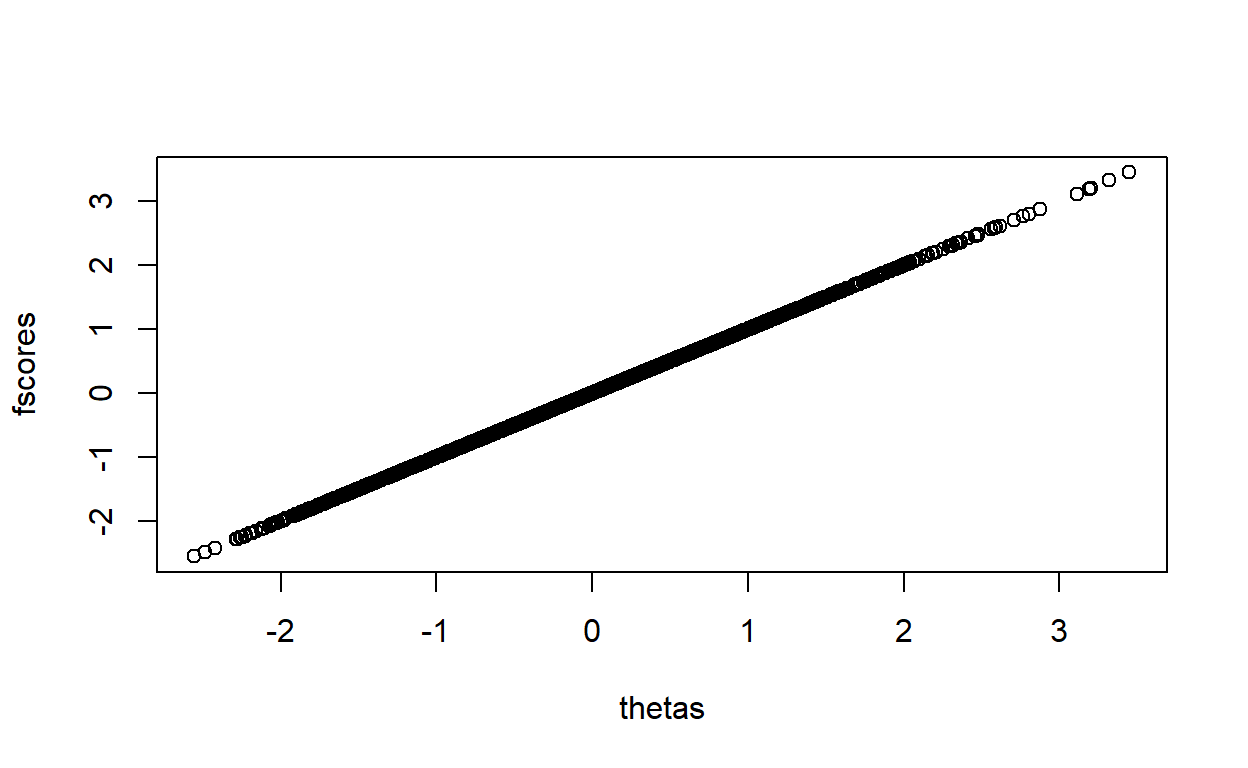### 2, Full-information Marginal Maximum Likelihood Estimation This approach is fully described in two papers, Wang and Zeng (1998) and Shojima(2005). Wang and Zeng (1998) is a classical implementation of EM algorithm. However, Shojima(2005) is a really interesting paper as he derives the closed-form equation for the marginal likelihood, so there is no integration needed for the E-step. When I was first interested in this model, I created an R package, EstCRM, that implements both approaches. Below is a code using this package to fit the model. require(EstCRM) CRM <- EstCRMitem(wpm[,1:3], max.item=c(250,250,250), min.item=c(0,0,0), max.EMCycle=500, converge=.01, type="Wang&Zeng", BFGS=TRUE) CRM$param
         [,1]     [,2]      [,3]
[1,] 3.090524 1.598122 0.7918418
[2,] 4.896383 1.192894 0.9677061
[3,] 4.470768 1.336568 0.9575835

Here it is! Again, we get almost identical estimates for item parameters. It is also possible to compute $$\theta$$ and compare them from the person paramet